# CBSE Sample Papers for Class 12 Maths Solved 2016 Set 5

0
1004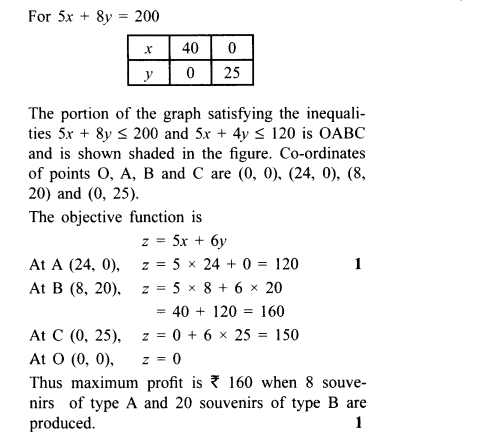SECTION — A

Question numbers 1 to 6 carry 1 mark each.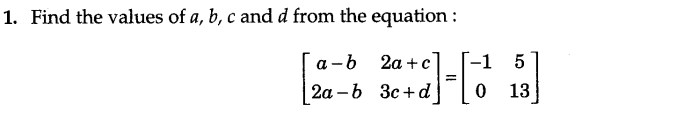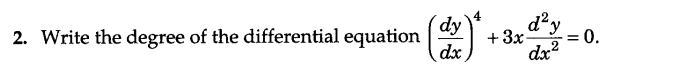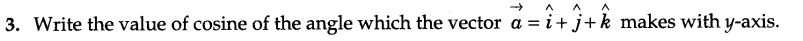4.Write the distance of a point P(a, b, c) from x-axis.

5.Write two different vectors having same direction, but different magnitude.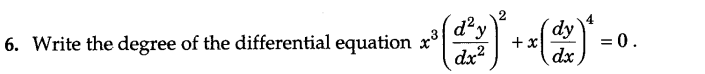SECTION — B

Question numbers 7 to 19 carry 4 marks each.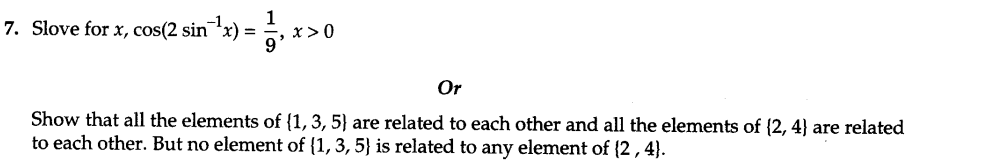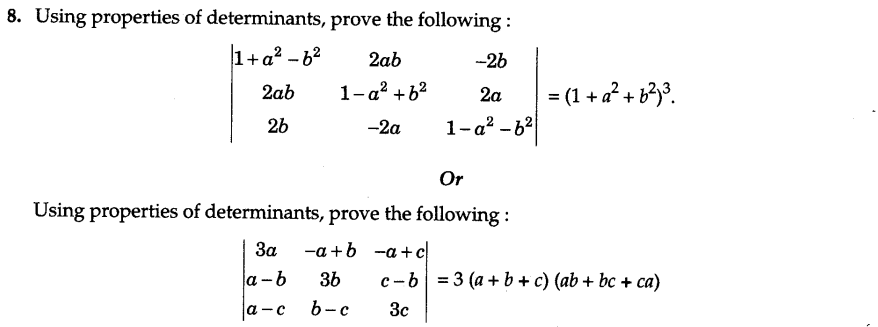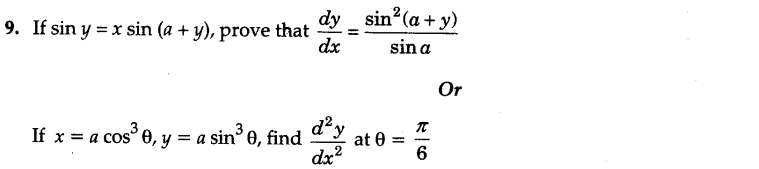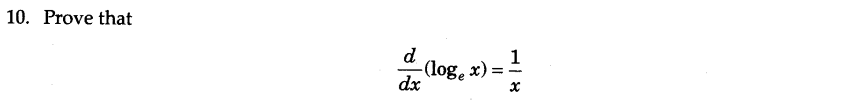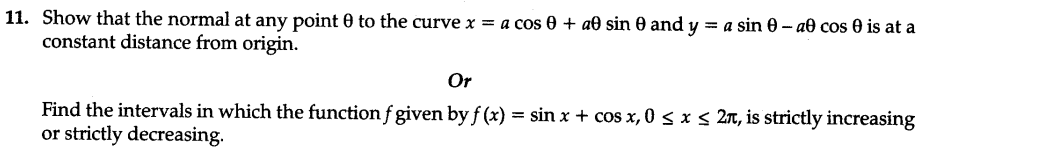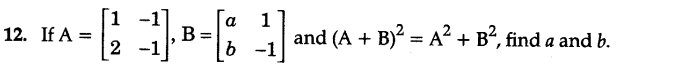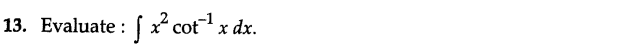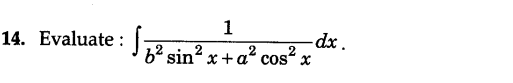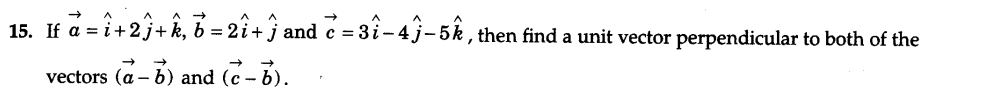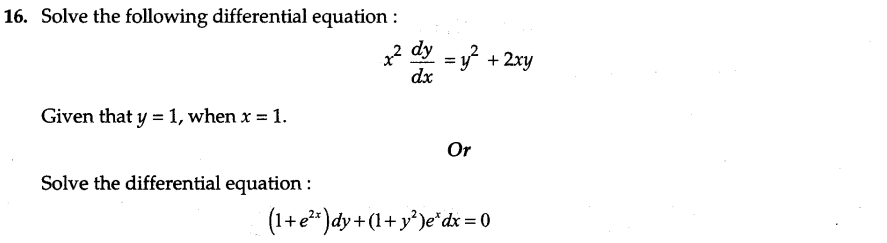17.Find the direction cosines of the vector joining the points A (1,2, -3) and B (-1, -2,1), directed from A to B.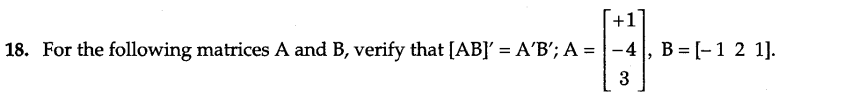19.In a class having 60% boys, 5% of the boys and 10% of the girls have an I.Q. of more than 150. A student is selected at random and found to have an I.Q. of more than 150. Find the probability that the selected student is a boy.

SECTION — C

Question numbers 20 to 26 carry 6 marks each.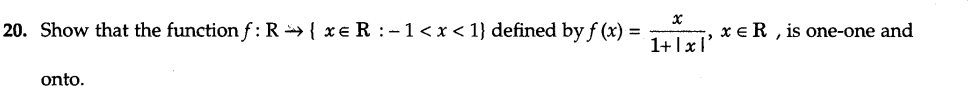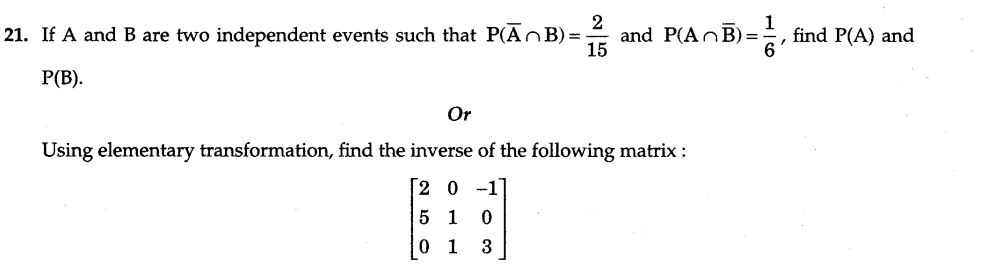22.A rectangle is inscribed in a semi-circle of radius r with one of its side on the diameter of the semi­circle. Find the maximum area of the rectangle.
Or
Show that the right circular cone of least curved surface and given volume has an altitude equal to root 2 times the radius of the base.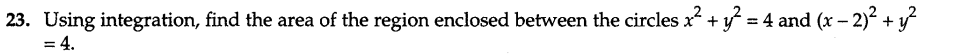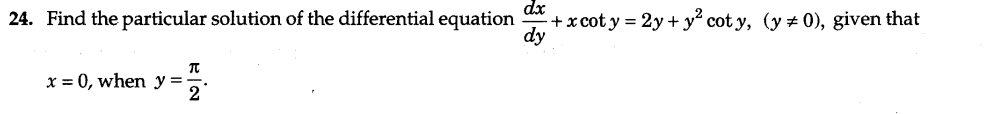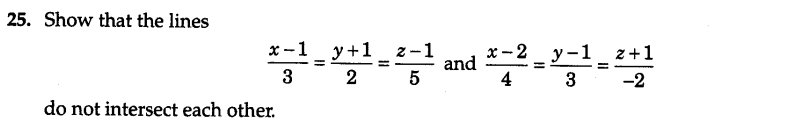26.A company manufactures two types of novelty souvenirs made of plywood. Souvenirs of type A require 5 minutes each for cutting and 10 minutes each for assembling. Souvenirs of type B require 8 minutes each for cutting and 8 minutes each for assembling. There are 3 hours 20 minutes available for cutting and 4 hours for assembling. The profit is Rs 5 each for type A and Rs 6 each for type B souvenirs. How many souvenirs of each type should the company manufacture in order to maximize the profit ?

SECTION — A

Question numbers 1 to 6 carry 1 mark each.Ans.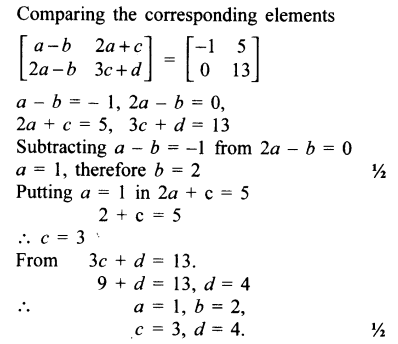Ans.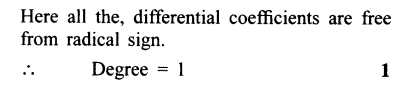Ans.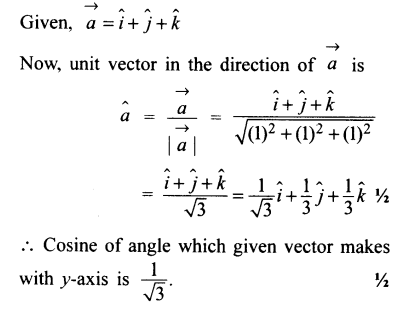4.Write the distance of a point P(a, b, c) from x-axis.
Ans.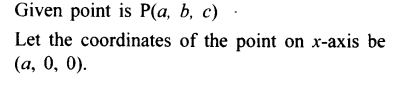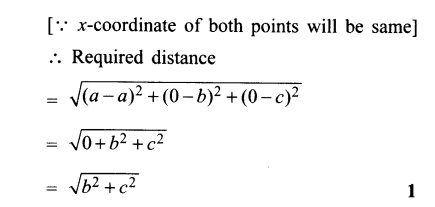5.Write two different vectors having same direction, but different magnitude.
Ans.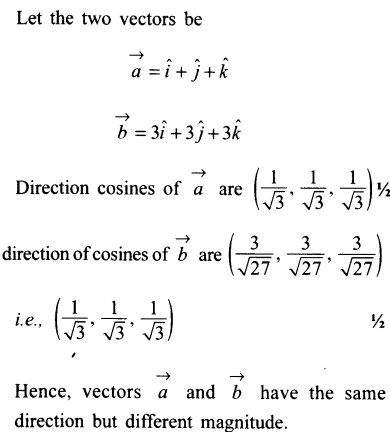Ans.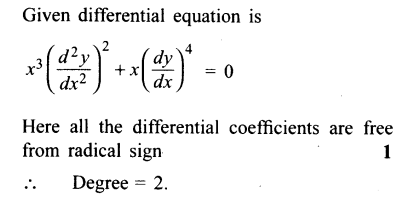SECTION — B

Question numbers 7 to 19 carry 4 marks each.Ans.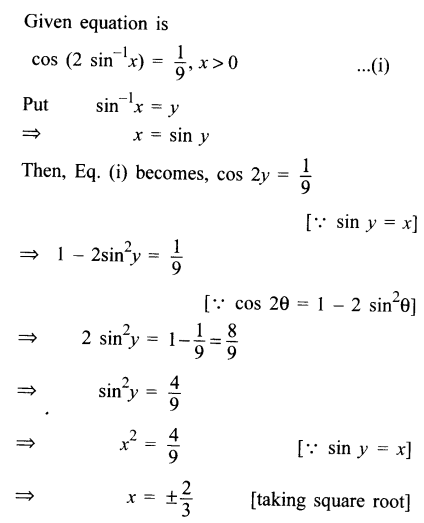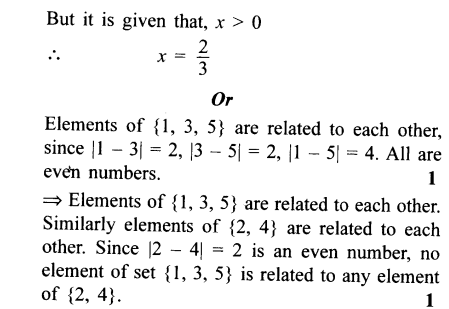Ans.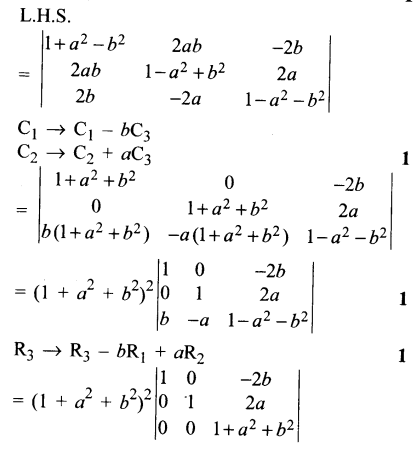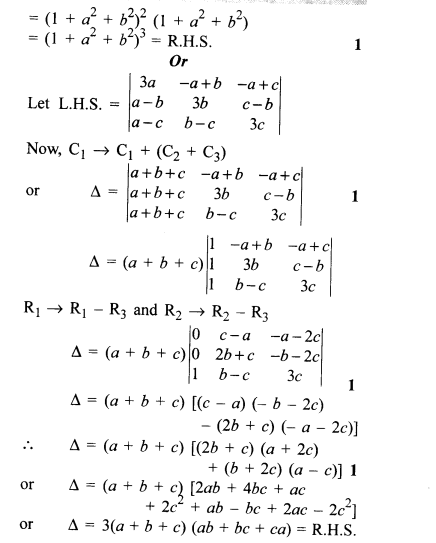Ans.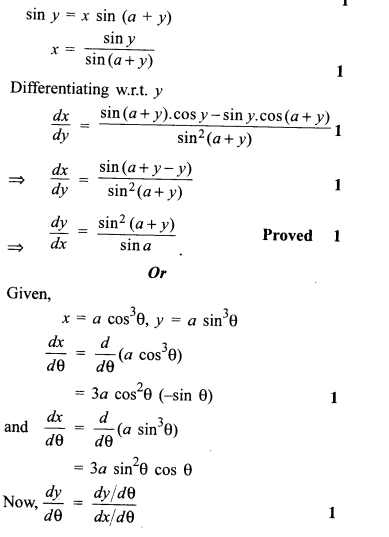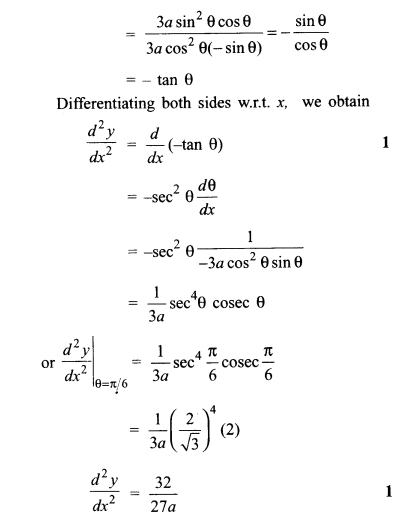Ans.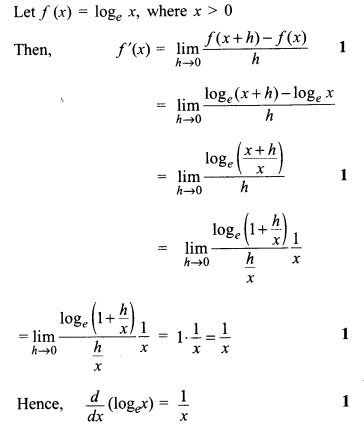Ans.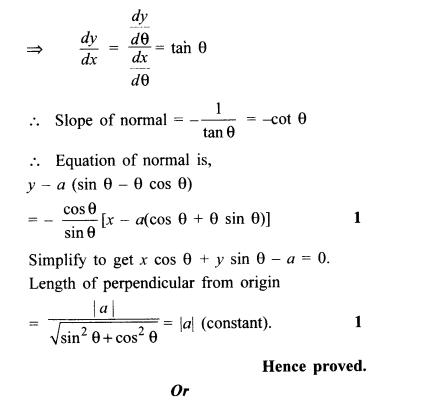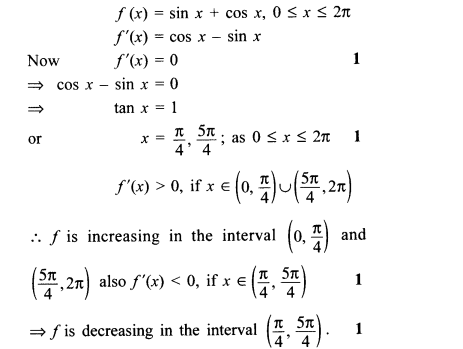Ans.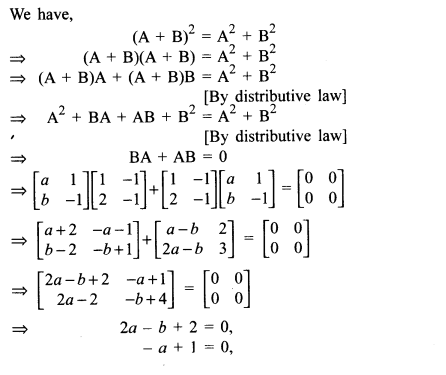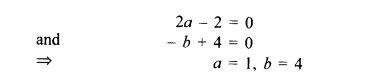Ans.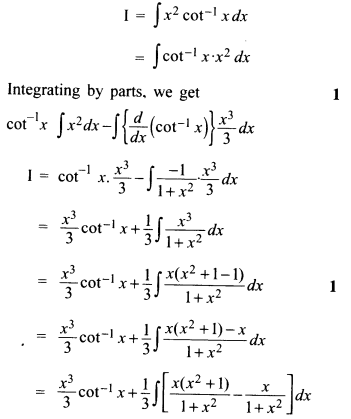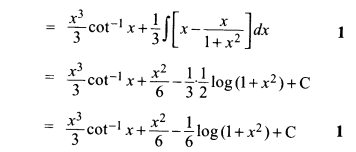Ans.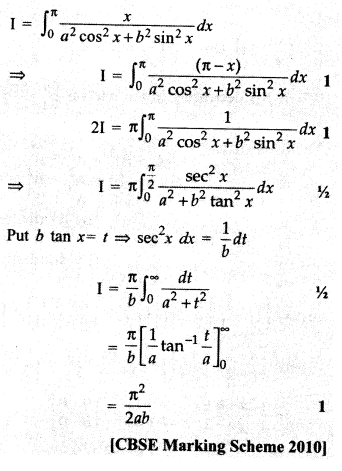Ans.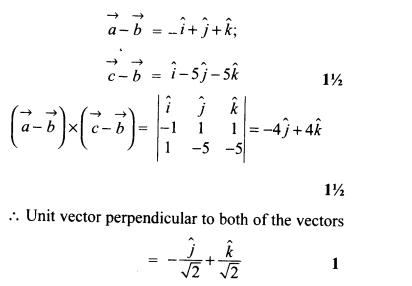Ans.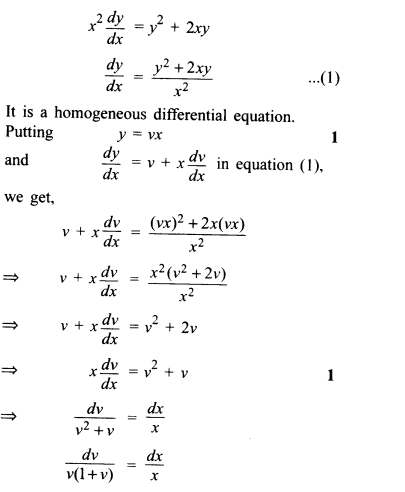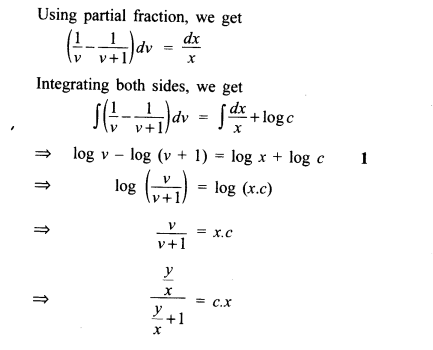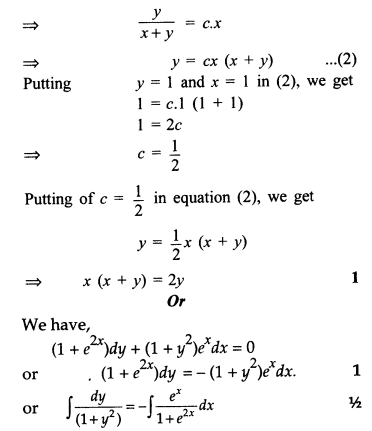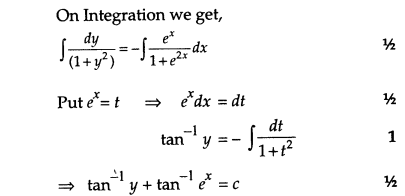17.Find the direction cosines of the vector joining the points A (1,2, -3) and B (-1, -2,1), directed from A to B.
Ans.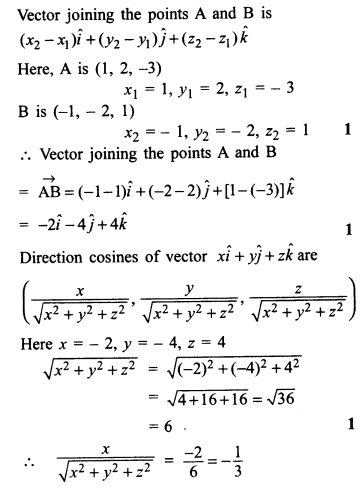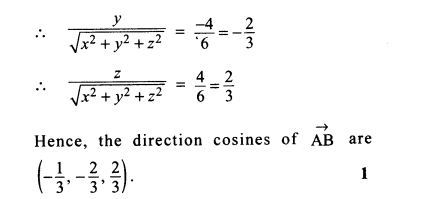Ans.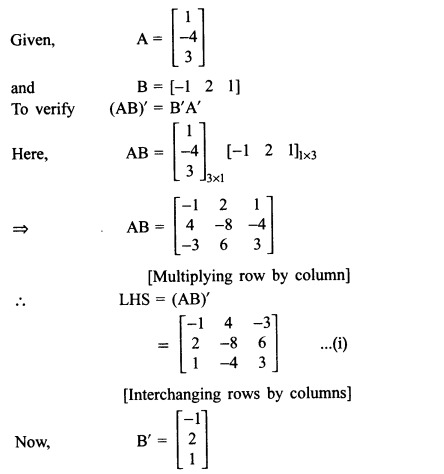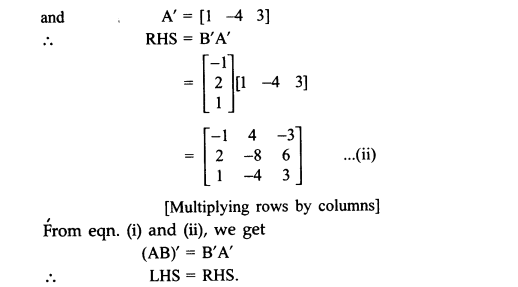19.In a class having 60% boys, 5% of the boys and 10% of the girls have an I.Q. of more than 150. A student is selected at random and found to have an I.Q. of more than 150. Find the probability that the selected student is a boy.
Ans.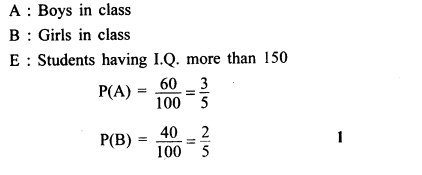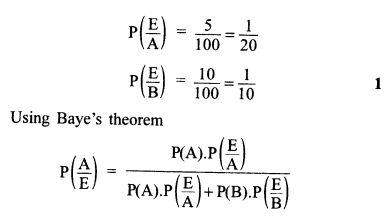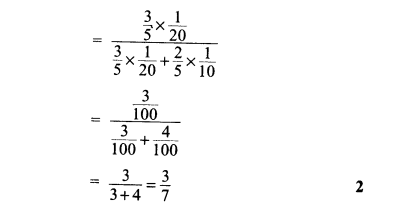SECTION — C

Question numbers 20 to 26 carry 6 marks each.Ans.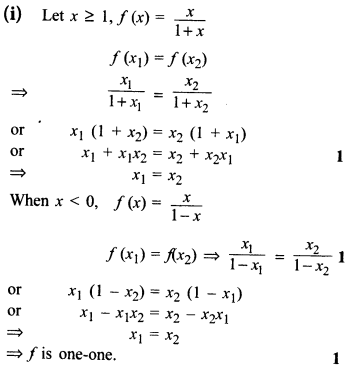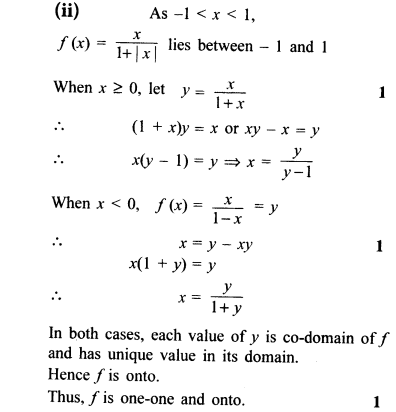Ans.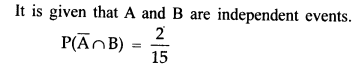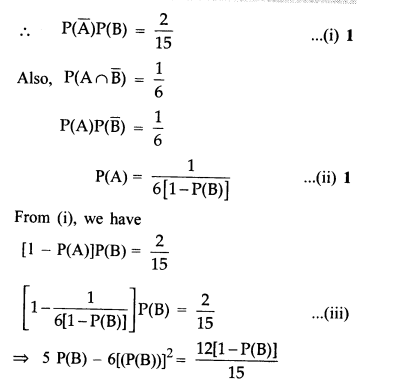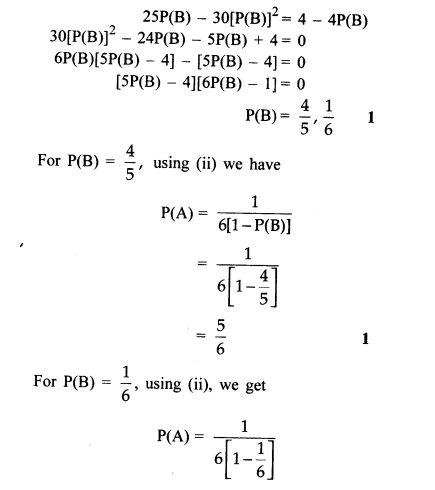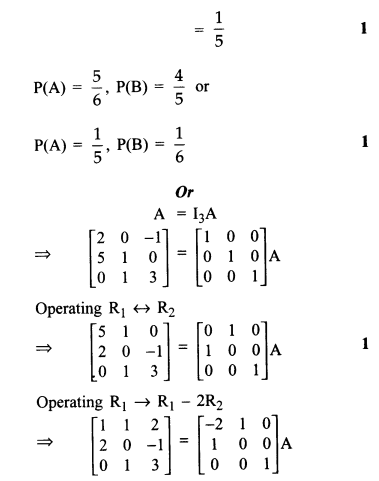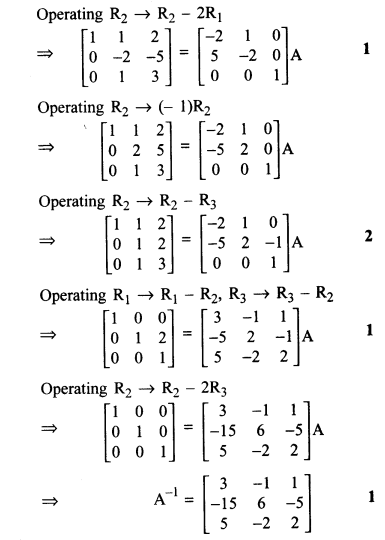22.A rectangle is inscribed in a semi-circle of radius r with one of its side on the diameter of the semi­circle. Find the maximum area of the rectangle.
Or
Show that the right circular cone of least curved surface and given volume has an altitude equal to root 2 times the radius of the base.

Ans.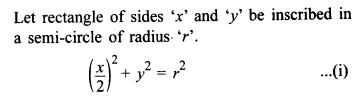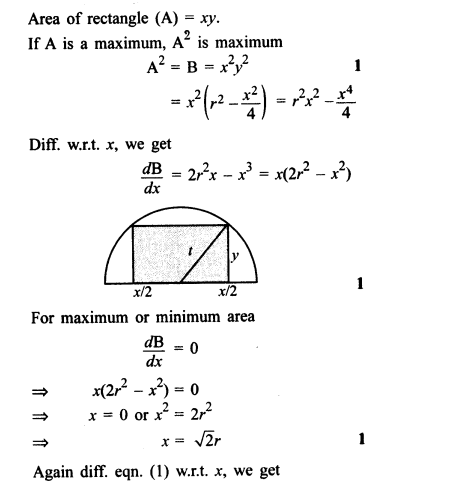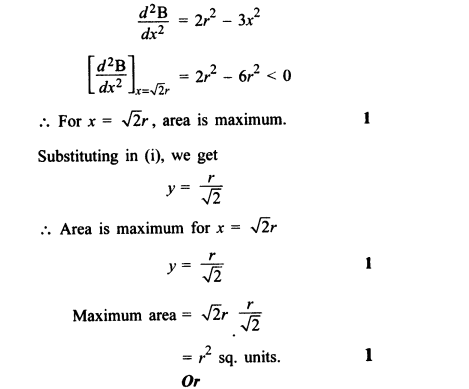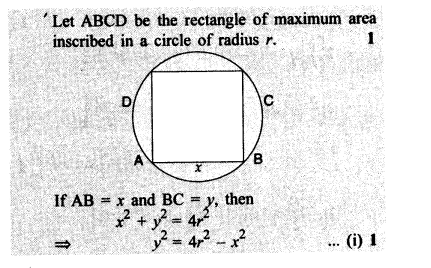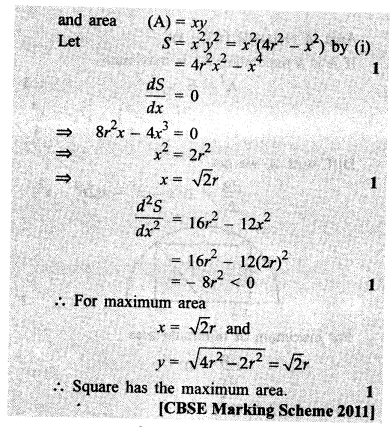Ans.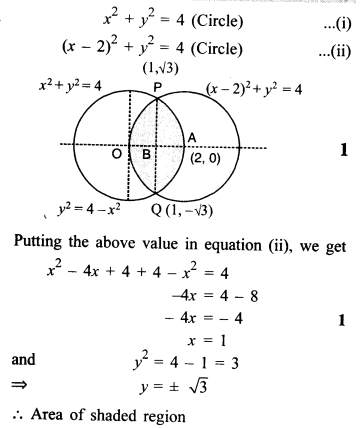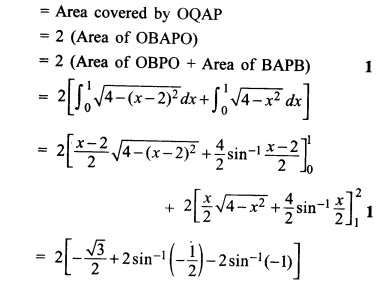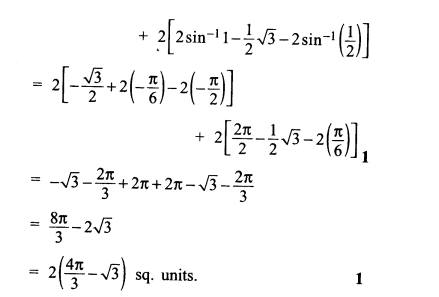Ans.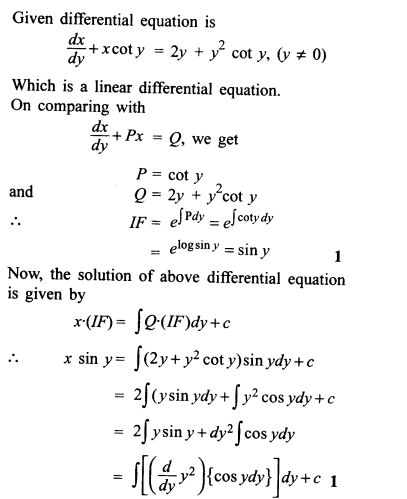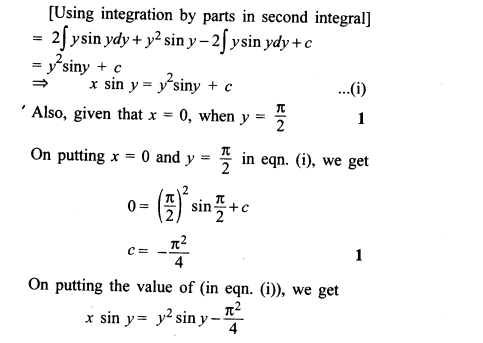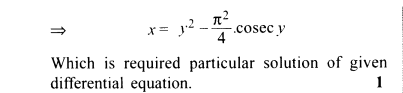Ans.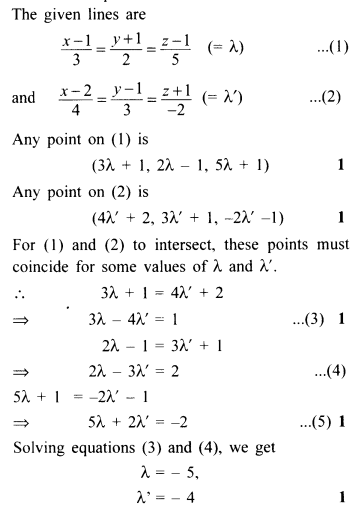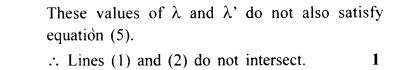26.A company manufactures two types of novelty souvenirs made of plywood. Souvenirs of type A require 5 minutes each for cutting and 10 minutes each for assembling. Souvenirs of type B require 8 minutes each for cutting and 8 minutes each for assembling. There are 3 hours 20 minutes available for cutting and 4 hours for assembling. The profit is Rs 5 each for type A and Rs 6 each for type B souvenirs. How many souvenirs of each type should the company manufacture in order to maximize the profit ?
Ans.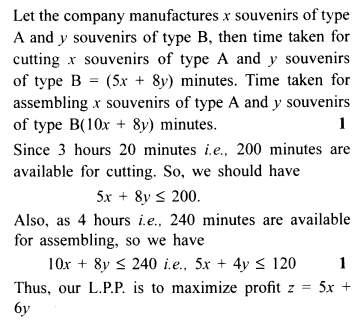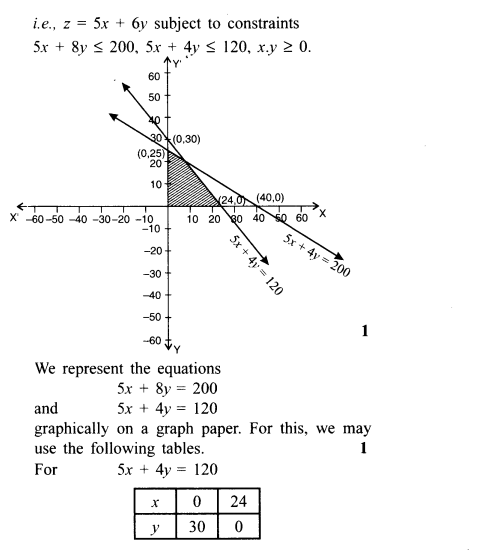Previous articleCBSE Sample Papers for Class 12 Maths Solved 2016 Set 4